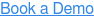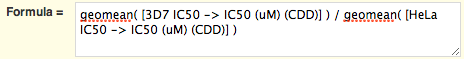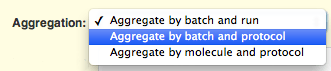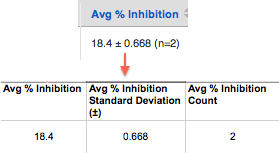June 27, 2014

# CDD Vault Update: Calculations Using Multiple Protocols

The flagship feature of this release is the ability to perform calculations using results from multiple assays. This allows you to calculate the selectivity index and similar metrics.

#### Calculations between protocols – part of CDD Vision

The readout definitions you use in calculations can now come from multiple protocols. Like all calculations in CDD Vision, results are dynamically updated in real time as you add new data, mark outliers, or fix errors. Here is the formula you would use to calculate the selectivity index, which compares potency to cytotoxicity.In order to compute meaningful results when a compound has been tested more than once, aggregated (average or median) values must be used. But don't worry: the application will help you with this.

#### Formula weight added to calculations – part of CDD Vision

Batch formula weight, which is calculated from the molecular weight plus the salt and hydrate data, has been added to the list of chemical properties you can use in your formulas.

#### Sum function – part of CDD Vision

In addition to aggregation functions such as mean and median, you can now calculate the sum of all values for a molecule or batch. This can provide a lightweight inventory mechanism, by subtracting the sum of all debits from an initial amount.

#### Batch-protocol aggregation scope

A new aggregation level for average and median calculations is shown in a drop-down below the formula field. With this scope, a value is calculated for each batch of a molecule, across all runs of the current protocol. There will be as many average values as there were tested batches of a molecule in a protocol. This is the best option to keep track of batch-to-batch variation.#### Better export of aggregated values

Calculated aggregate readouts that are exported from CDD Vault are now returned in three separate columns for ease of manipulation: value (average or median), standard deviation, and number of samples (n).## Other posts you might be interested in

View All Posts